# AP Board 8th Class Maths Solutions Chapter 11 Algebraic Expressions Ex 11.5

AP State Syllabus AP Board 8th Class Maths Solutions Chapter 11 Algebraic Expressions Ex 11.5 Textbook Questions and Answers.

## AP State Syllabus 8th Class Maths Solutions 11th Lesson Algebraic Expressions Exercise 11.5Question 1.
Verify the identity (a + b)2 ≡ a2 + 2ab + b2 geometrically by taking
(i) a = 2 units, b = 4 units
(ii) a = 3 units, b = 1 unit
(iii) a = 5 units, b = 2 unit
Solution:
(i)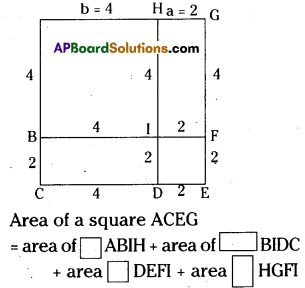= 4 × 4 + 4 × 2 + 2 × 2 + 4 × 2
= 16+ 8 + 4 + 8 = 36 sq.units
[∵ (2 + 4)2 = 62 = 36](ii)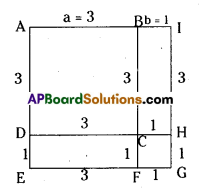Area of a square AEGI
= area of square ABCD + area of rectangle CDEF + area of square CFGH + area of rectangle BIHC.
= 3 × 3 + 3 × 1 + 1 × 1+3 × 1
= 9 + 3 + 1 + 3
= 16 sq. units
[∵ (3 + 1)2 = 42 = 16]

(iii)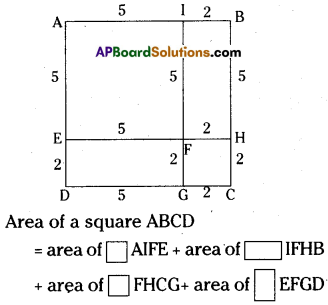= 5 × 5 + 2 × 5 + 2 × 2 + 5 × 2
= 25 + 10 + 4 + 10
= 49 sq.units
[∵ (5 + 2)2 = 72 = 49]Question 2.
Verify the identity (a – b)2 ≡ a2 – 2ab+ b2 geometrically by taking
(i) a = 3 units, b= 1 unit
(ii) a = 5 units, b = 2 units
Solution:
(i)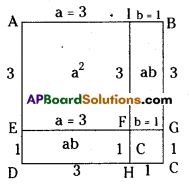Area of AIFE + Area of FGCH = (a – b)2 = a2 – 2ab + b2 [area of AIFE – area of IBGF – area of EFHD + area of FGCH]
= 3 × 3 – 1 × 3 – 3 × 1 + 1 × 1
= 9 – 3 – 3 + 1 = 4
∴ (a – b)2 = 4 sq. units
[∵ (3 – 1 )2 = 22 = 4]

ii)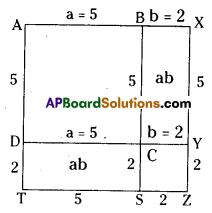∴ (a – b)2 = a2 – 2ab + b2
Area of ABCD + Area of CYZS = a2 – 2ab + b2
area of ABCD – area of BXYC – area of DCST + area of CYZS
=5 × 5 – 2 × 5 – 2 × 5 + 2 × 2
= 25 – 10 – 10 + 4
= 9 sq.units
[∵ (5 – 2)2 = (3)2 = 9]Question 3.
Verify the identity(a + b)(a – b) ≡ a2 – b2 geometrically by taking
(i) a = 3 units, b = 2 units
(ii) a = 2 units, b = 1 unit
Solution:
i)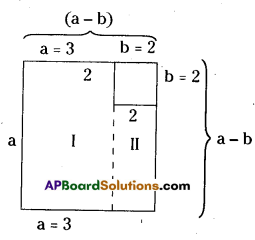a2 – b2 = Area of Fig I. + Area of Fig II
= a(a – b) + b(a – b)
= (a – b) (a + b)
= 3 × 3 – 2 × 2
a2 – b2 = 9 – 4= 5sq . units
[ ∵ 32 – 22 = 9 – 4 = 5]ii)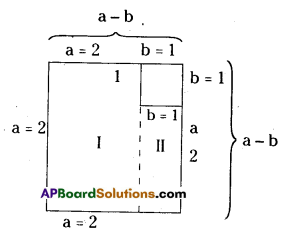a2 – b2 = Area of Fig I. + Area of Fig II
= a(a – b) + b(a – b)
= (a + b) (a – b)
=(2 + 1)(2 – 1)
= 3 × 1 = 3
a2 – b2 = 3 sq. units
[∵ (22 – 12) = 4 – 1 = 3]